It takes only one rogue voltage wave to kill a power distribution network (PDN) in high-speed digital designs. Rogue voltage waves occur when ripples from more than one PDN resonance line up in phase to create an excessive rogue wave . Flat impedance optimization before layout lowers the risk of rogue waves occurring in your design. Power integrity (PI) engineers use target impedance to design power delivery across a broad frequency range. Target impedance is a simple estimate using the maximum allowed ripple divided by the maximum expected current step load. For example, in a field-programmable gate array (FPGA) where the maximum ripple is 60 mV and the maximum current step load is 5 A, the target impedance (Z) is 12 mOhm (see Figure 1).

Achieving this target impedance across a broad frequency range requires a power supply for the low frequencies and decoupling capacitors for the higher frequencies. The challenge is to get the best performance at the lowest cost. Flat impedance design makes that possible. A PDN designed for flat impedance uses the minimum number of capacitors to achieve a target impedance while avoiding high Q resonances that can cause rogue voltage waves. Reducing part count has the added benefit of higher reliability with fewer solder joints.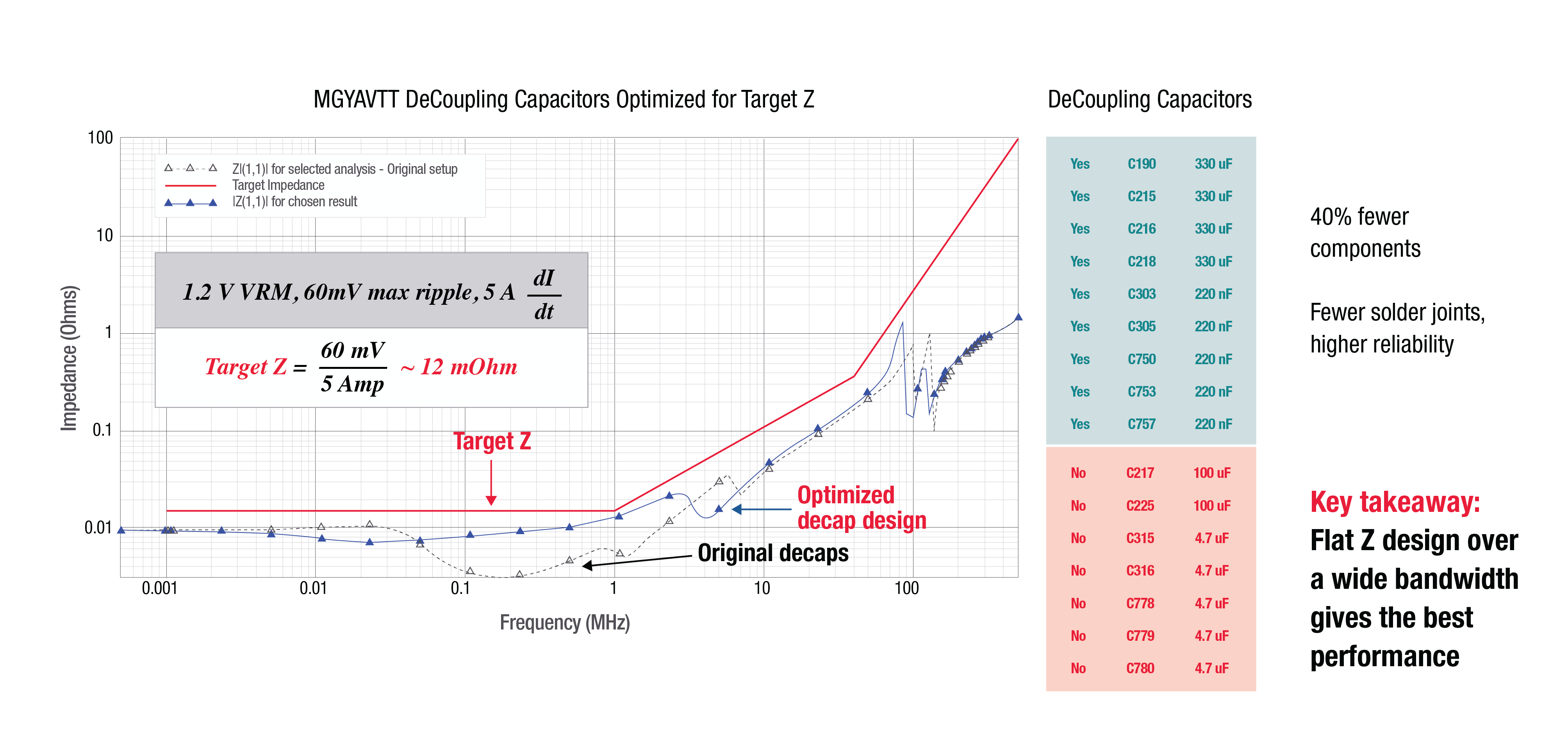Figure 1:  In this FPGA example, 60 mV ripple divided by 5 A gives a target impedance of 12 mOhms.

Figure 1 shows a plot of the impedance versus frequency for a 1.2 V power rail with the target impedance mask in red . The Target Z in red shows the flat region where the power supply and printed circuit board (PCB) PDN must deliver power to the load. The first breakpoint, around 1 MHz, shows the frequency at which the internal package/die capacitance is starting to provide power to the load. The second breakpoint shows that the spectral content of the load is rapidly diminishing with frequency. Higher PDN impedances are allowed as di/dt goes to zero.

The PI designer has control over only the range of frequencies covered by the voltage regulator module (VRM) and the PCB decoupling capacitors. This is where flat impedance design can improve performance and lower cost.

The challenge in selecting decoupling capacitors is that at mOhms of target impedance, the PCB routing parasitics, and component package parasitics are significant. The decoupling capacitor recommendations leveraged from a data sheet may no longer be accurate or provide the best outcome. Selecting the wrong capacitors can result in unwanted resonances and the potential for rogue waves.

### How Rogue Waves Occur

To avoid the resonances that lead to rogue waves on the power rail, it is important to understand how rogue waves occur (see Figure 2). A common source of ringing on the power rail comes from the parallel resonance of an inductor (L) with a capacitor (C). The inductor stores energy in the magnetic field with the phase of the voltage leading the current. A capacitor stores energy in the electric field with the voltage phase lagging the current. Like a swing with energy oscillating between potential and kinetic, the current in the inductor and capacitor moves back and forth.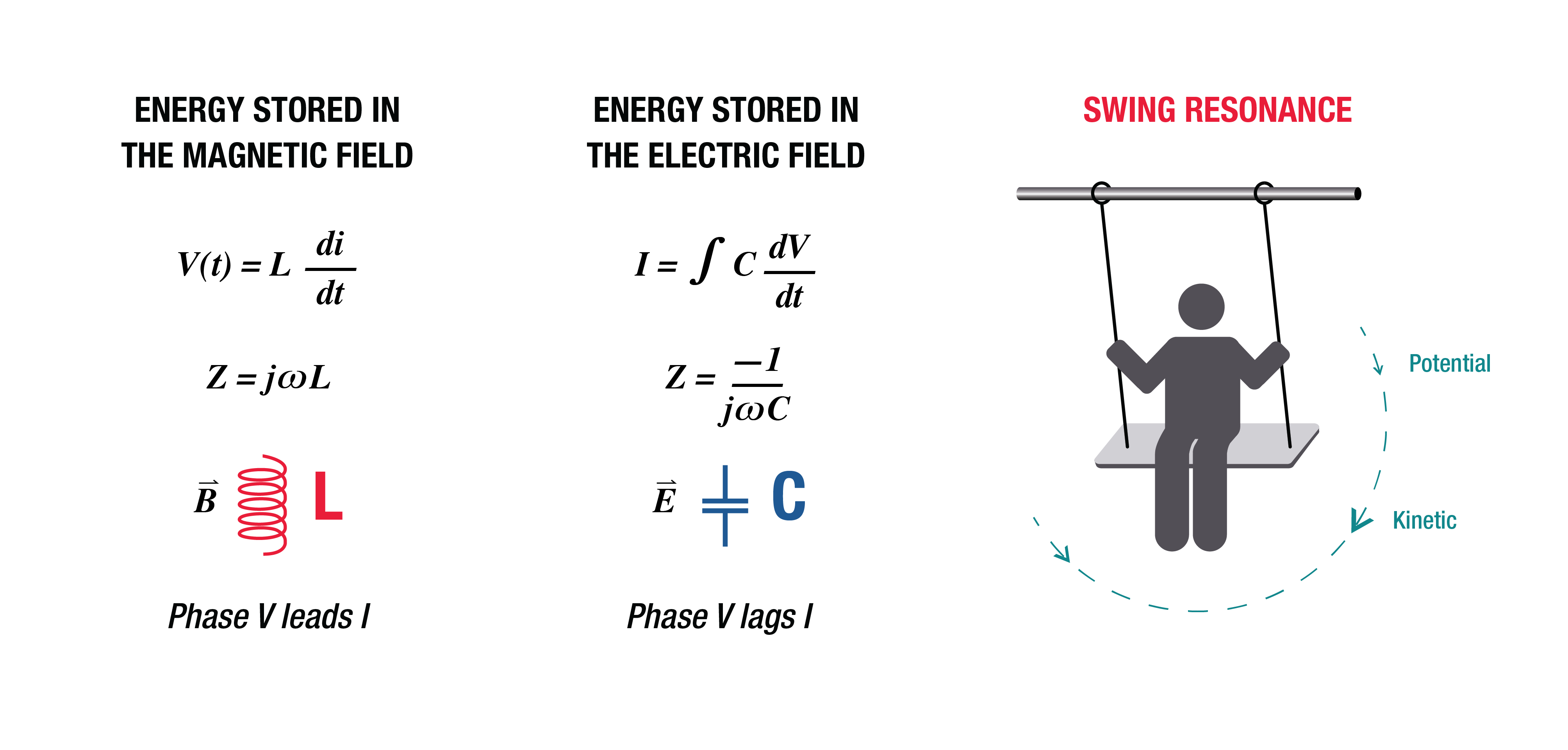Figure 2:  Parallel resonance of inductors and capacitors leads to ringing on the power rail.

The schematic (see Figure 3) illustrates the root cause of parallel L/C ringing on the power rail. A simple series R-L model of the VRM shows how the active inductance of the VRM is in parallel with the large bulk capacitors. The bulk capacitor also has internal series package inductance in parallel with the smaller decoupling capacitors. In the simple SPICE-type simulation of the bottom left schematic, the sweep of a 1 A AC current load excites the parallel L and C to generate the impedance versus frequency plot on the right.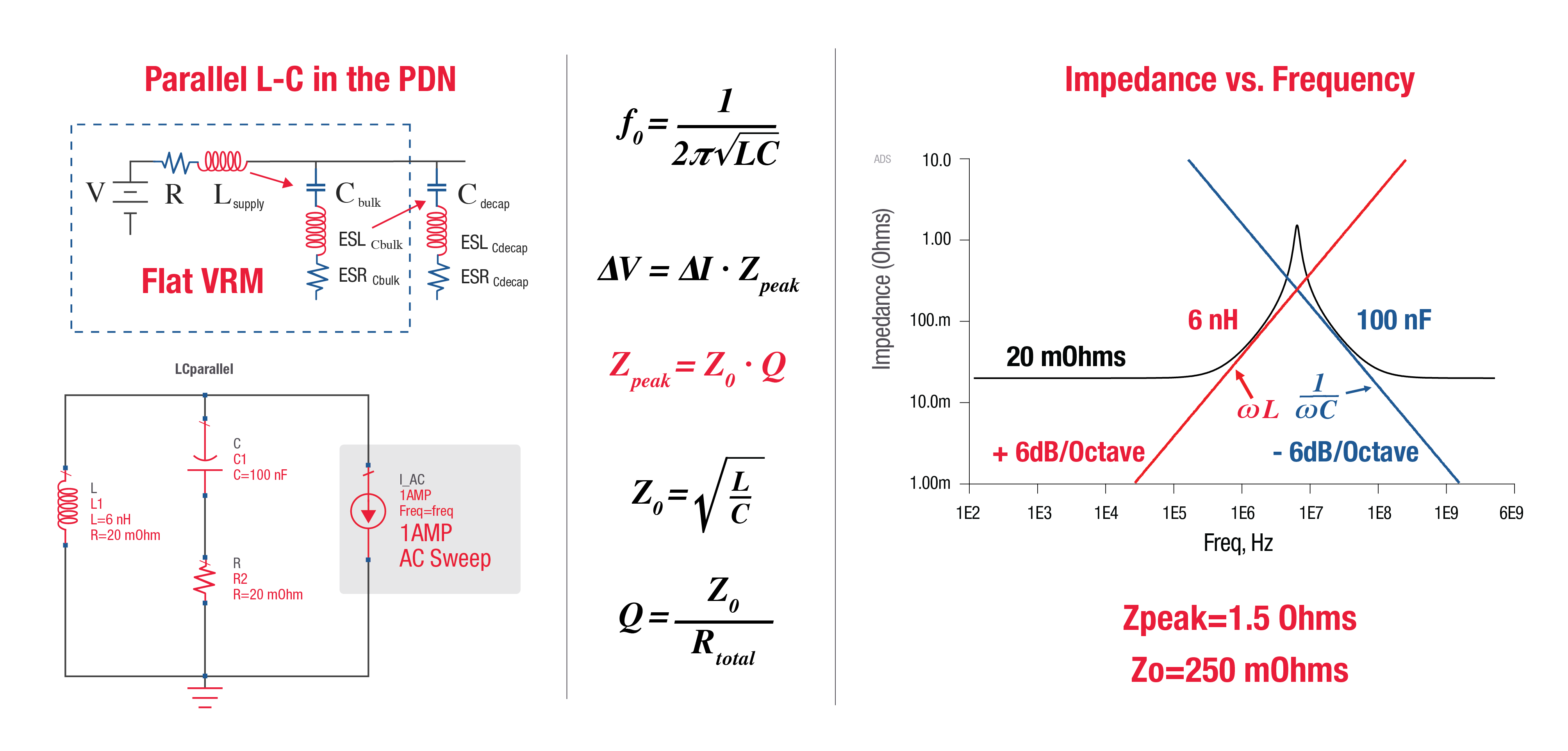Figure 3:  Simulation of inductance resonating with the parallel decoupling capacitance predicts ringing on the power rail at the frequency of the 1.5 Ω impedance peak.

The simulation clearly shows a peak impedance at the LC resonant frequency. A few basic electrical equations characterize the peak frequency and show that the maximum impedance depends on the sharpness or Q of the resonance. Increasing the real resistance in series with the L and C reduces Q. This series resistance is important in designing for flat impedance. Resistors that help reduce the resonant Q are known as dampening resistors or snubber resistors.

The impedance plot on the right in Figure 3 shows how the 20 mOhm resistance of the VRM dominates at low frequency. As the frequency increases, the active inductance of the VRM takes effect with an upward 6nH inductive slope. The VRM inductance then resonates with the 100nF downward slope of the bulk capacitor to give a peak impedance of 1.5Ω. This impedance peak indicates a resonance in the PDN that, when excited by a forcing function at that frequency, results in a voltage ripple that is Q times larger than the step response. Exciting multiple PDN resonant frequencies can result in rogue voltage waves that exceed the design margins.

### Adding the Right Decoupling Capacitors for Flat Impedance

To understand how to select the correct decoupling capacitors, look at the design of a PDN when the VRM connects to the package/die model without decoupling capacitors (see Figure 4). The plot on the left shows the impedance versus frequency as seen by the load with the VRM series R-L model connected directly to the package/die behavioral model. In this case, the package die simulation model was an S-parameter data file from the vendor.

The original series R-L model is the dashed black trace. The dashed red trace is the package die S-parameter model. The blue trace is the impedance seen at the load when connecting the two models together. The resistive behavior of the VRM is at low frequency. Since there are no decoupling capacitors, only the package/die model, the VRM output inductance in parallel with the package capacitance creates a high impedance resonance. At higher frequencies, the behavior of the package/die S-parameter model dominates the impedance at the load.

The log time-domain plot on the right shows the forced response at the peak in the impedance, causing a worst-case voltage ripple on the power rail. The peak impedance may be low enough to handle a single step, but the forced response at this frequency grows exponentially, showing a worst-case noise ripple. Eliminating the resonance requires the addition of decoupling capacitors.

.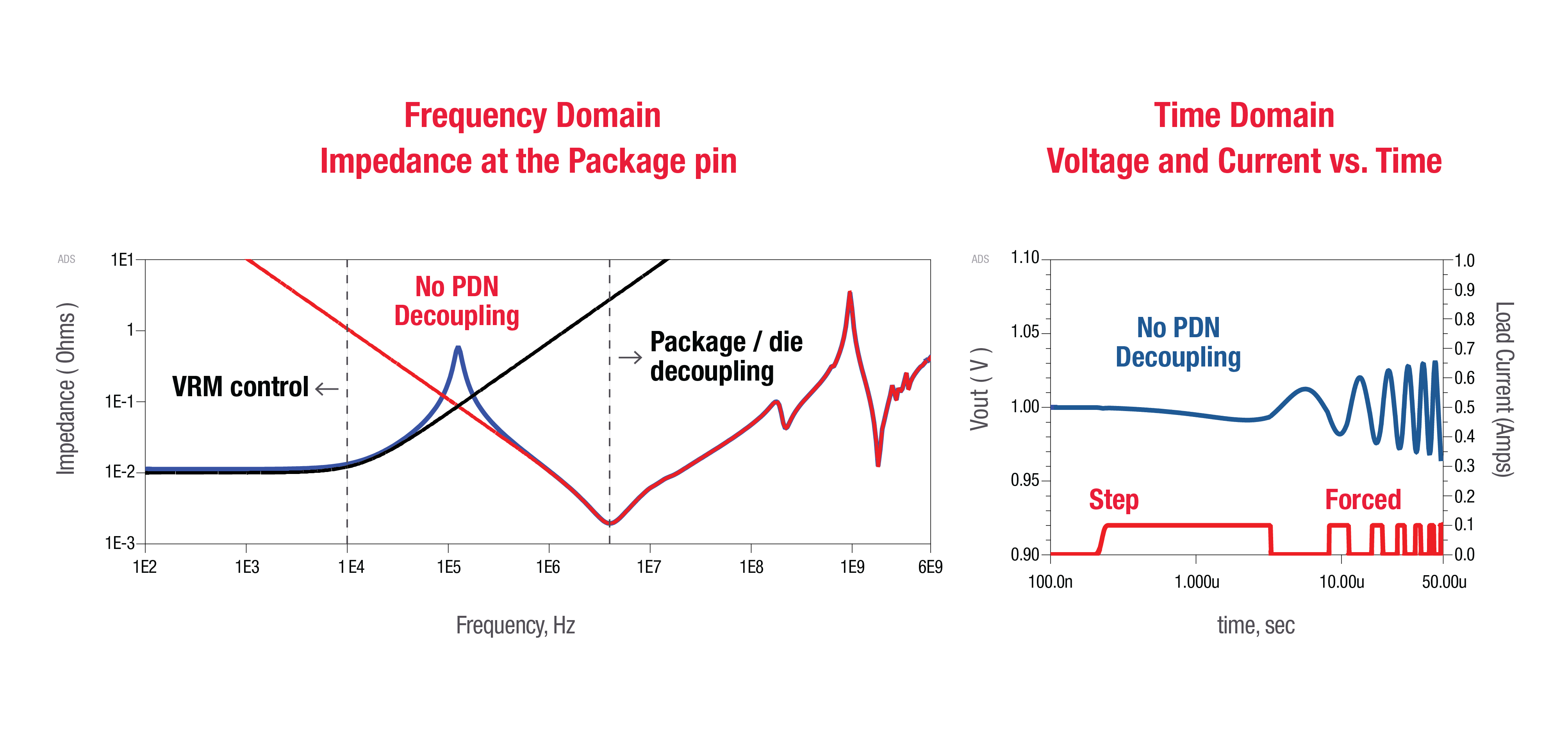Figure 4:  Parallel resonance in the VRM plus load causes an impedance peak without decoupling capacitors. Time domain simulation with a log time scale shows the excessive forced response ripple.

Adding a capacitor with a factor of 10 value, such as a 100uF capacitor leveraged from a previous design or data sheet recommendation, reduces the impedance peak (see Figure 5). The resulting time domain ripple is less at the original peak impedance frequency; however, notice that adding this incorrect capacitor has created two new impedance peaks shown by the blue trace.

The bulk 100uF capacitor is the wrong value for flat impedance, and the first peak shows it resonating with the VRM inductance. The second higher frequency peak is the capacitor’s equivalent series inductor resonating with the package/die capacitance. These two peaks are smaller but may still not meet the required target impedance, leaving the possibility for a rogue wave. Each peak requires additional capacitors to obtain the desired flat target impedance .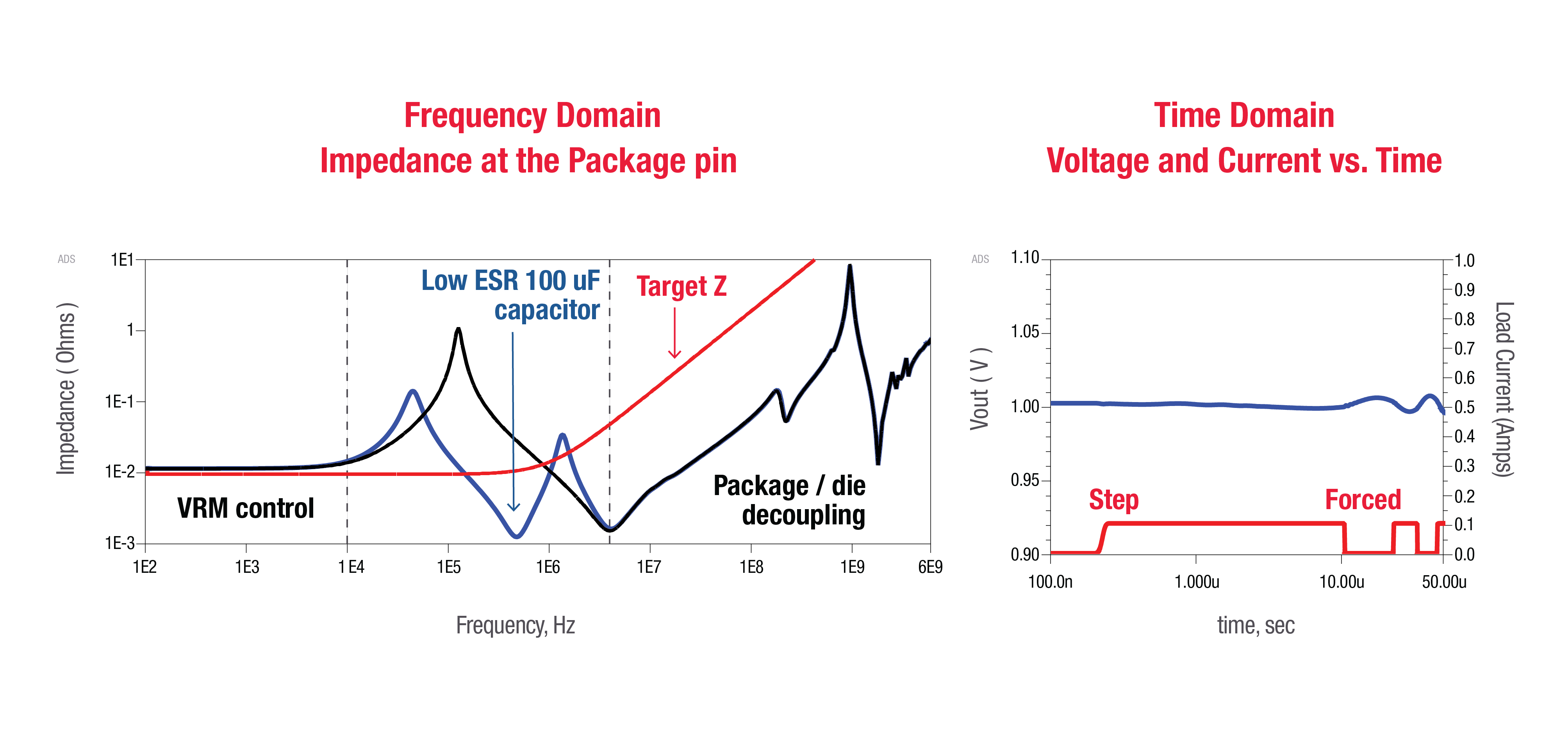.
Figure 5:  Selecting the wrong decoupling capacitor causes more parallel resonance impedance peaks.

Eventually, adding enough capacitors drives the impedance below the target design impedance. However, there is a better way that maximizes performance and minimizes cost. Instead of leveraging a suggested capacitor value from a data sheet or previous design, use the simple L over target Z squared equation to find the C required for flat impedance :By designing PDNs for flat target impedance, PI engineers can select the right value of capacitance and reduce part count (see Figure 6). The time domain plot on the right in Figure 6 shows that the flat impedance (solid blue trace) produces less ripple than the non-flat impedance. This simple design example is a good starting point. For a real system, however, the PCB and component parasitics are significant. PI engineers need a complete workflow to design and test the final product.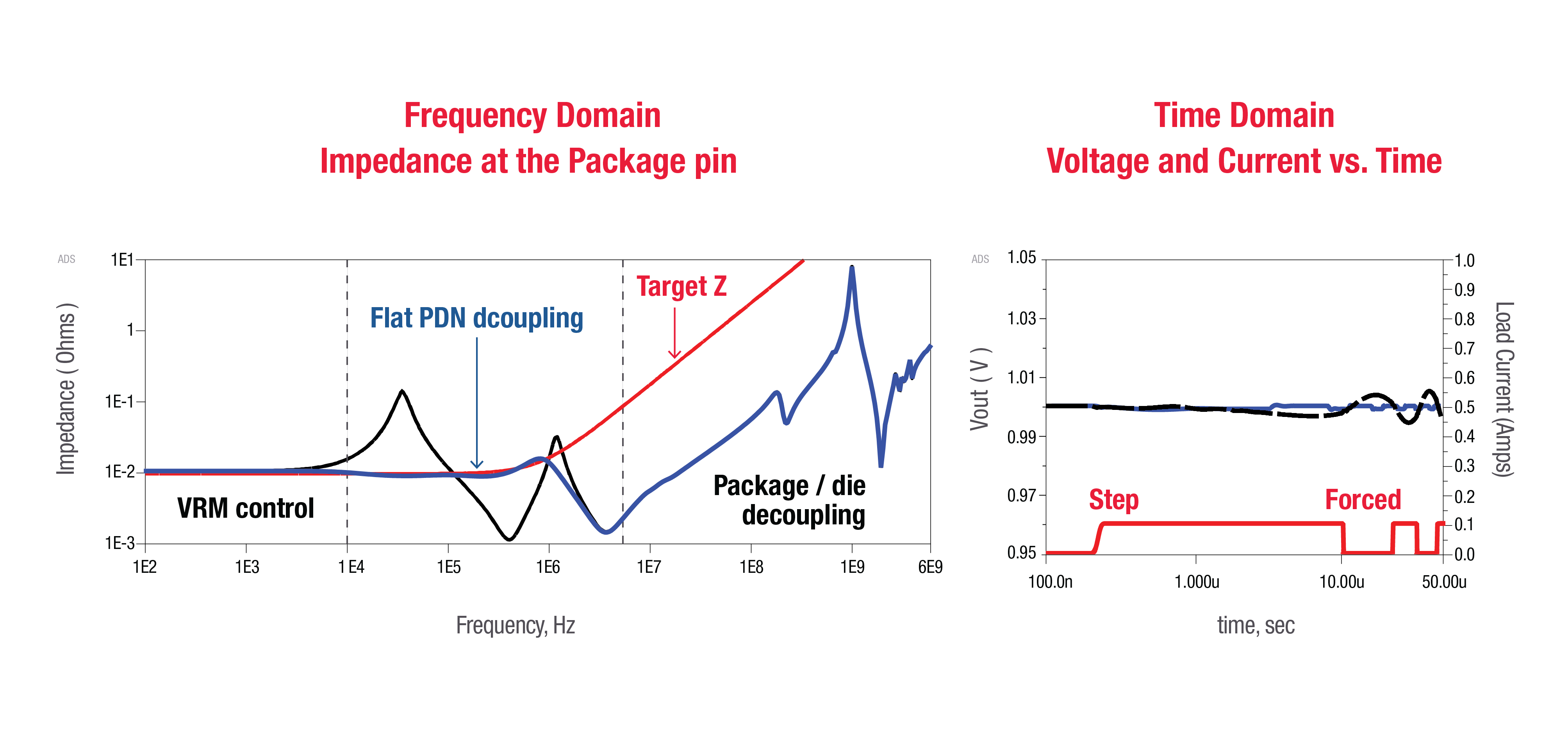Figure 6:  Flat impedance PDN design delivers maximum stability and minimum ripple (Blue Trace).

### Ensure Power Integrity with a Complete Workflow

In a full PI workflow, designers start with good models of the VRM, the decoupling capacitors, and the load . Often the best models are measurement-based, such as a state space average model of the VRM or a measured S-parameter model of the capacitor for the given application environment (see Figure 7). Next, designers perform pre-layout investigations to understand the power integrity challenges and provide layout guidelines.

Once the PCB layout is complete, designers run a full post-layout EM simulation and use the result to optimize the location and values of the decoupling capacitors. A final post-layout PI ecosystem simulation connects the high-fidelity VRM model with the PCB EM model, the optimized decoupling, and the package/die model to look at small signal AC impedance and load transients, as well as large signal DC-DC converter switching ripple. Measurement is the final step in the power integrity workflow. It verifies that the ripple is within specification, that load timing and amplitude jitter are acceptable, and that the design meets EMI/EMC standards.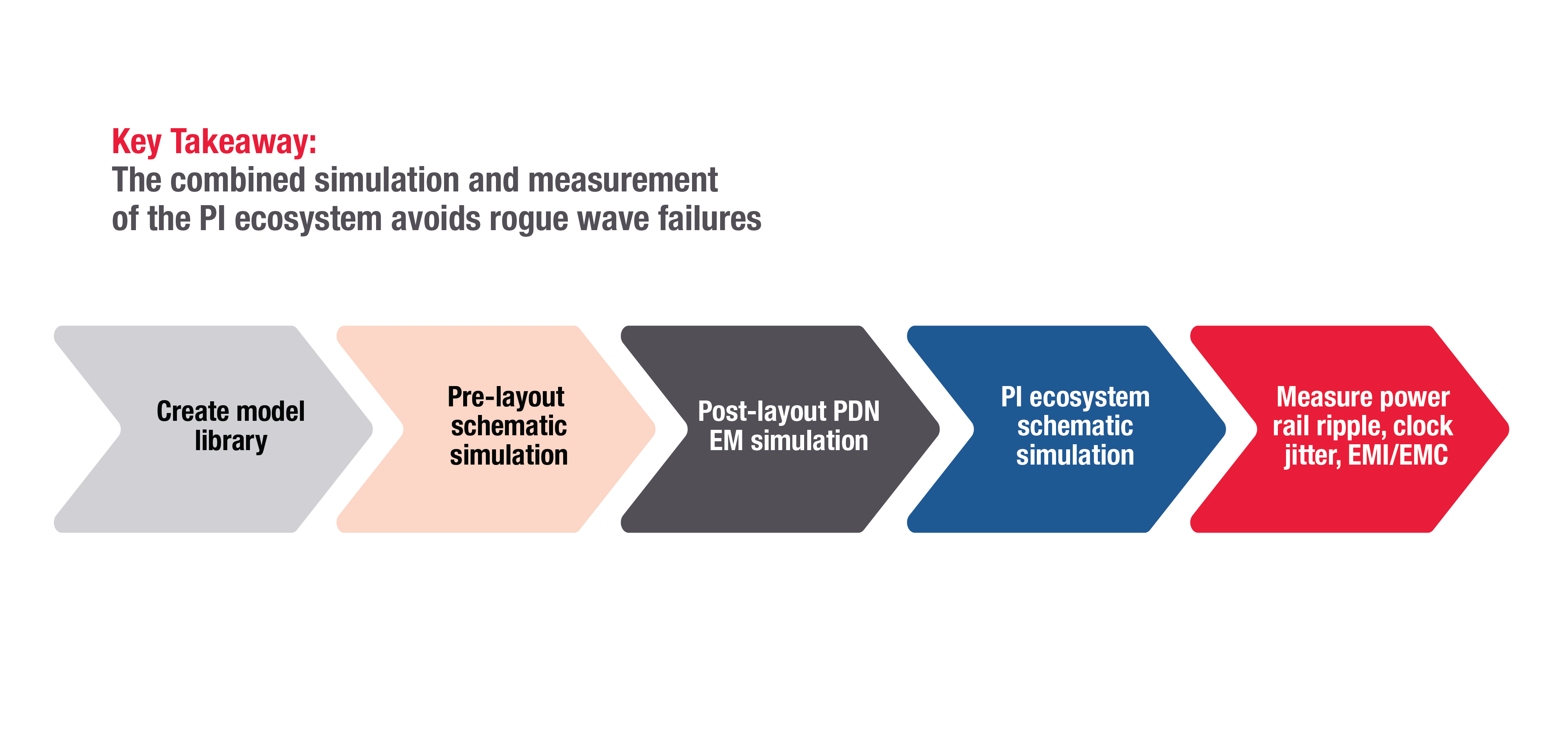.
Figure 7:  The PI workflow starts before layout and leverages simulation with measurement-based models to avoid late in the design cycle failures and to reduce product cost.

Too many designers leverage a previous design or a data sheet example and wait until measurement to debug. That is a risky and expensive way to design. Some engineers use free tools provided by power supply vendors such as LTspice, but fail to simulate the EM parasitics of the PCB and decoupling components. The PCB industry focuses on post-layout because this is usually where a manager must sign off on the design to verify that it is ready for fabrication. Starting at post-layout EM often leads to optimization of a bad design.

A better approach is to start with pre-layout to explore the right design space before going to layout and optimization. Combining simulation tools for power integrity and power electronics covers the whole PI ecosystem, from generation to delivery at the load. That gives designers a complete workflow to avoid failures late in the design cycle.

### References

 S. Sandler, “Target Impedance Limitations and Rogue Wave Assessments on PDN Performance” Signal Integrity Journal, March 19,2018.

 H. Barnes, J. Carrel, and S. Sandler, “Power Integrity for 32 Gb/s SERDES Transceivers,” in DesignCon, 2018.  L. Smith and E. Bogatin, Principles of Power Integrity for PDN Design. Prentice Hall, 2017.

 I. Novak, “Comparison of Power Distribution Network Design Methods” DesignCon 2006.

 H. Barnes and S. Sandler, “Decoupling Capacitor Optimization for Flat Z PCB Power Distribution Networks” 2018 IEEE Symposium on Electromagnetic Compatibility & Signal/Power Integrity (EMCSI).

 S. Sandler, “How to Design for Power Integrity” Keysight sponsored YouTube Video Series:  http://www.keysight.com/find/how-to-videos-for-pi.